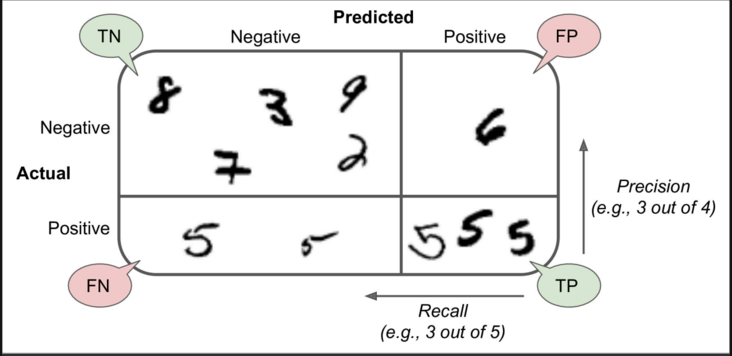# Confusion Matrix in Machine Learning

• Difficulty Level : Medium
• Last Updated : 18 Nov, 2021

Machine Learning – the study of computer algorithms that improve automatically through experience. It is seen as a subset of artificial intelligence.

Classification is the process of categorizing a given set of data into classes.
In Machine Learning(ML), you frame the problem, collect and clean the data, add some necessary feature variables(if any), train the model, measure its performance, improve it by using some cost function, and then it is ready to deploy.
But how do we measure its performance? Is there any particular feature to look at?
A trivial and broad answer would be to compare the actual values to the predicted values. But that does not solve the issue.
Let us consider the famous MNIST dataset and try to analyze the problem.

## Python3

 `# Importing the dataset.``from` `sklearn.datasets ``import` `fetch_openml``mnist ``=` `fetch_openml(``'mnist_784'``, version``=``1``)` `# Creating independent and dependent variables.``X, y ``=` `mnist[``'data'``], mnist[``'target'``]` `# Splitting the data into training set and test set.``X_train, X_test, y_train, y_test ``=` `X[:``60000``], X[``60000``:], y[:``60000``], y[``60000``:]` `"""``The training set is already shuffled for us, which is good as this guarantees that all``cross-validation folds will be similar.``"""` `# Training a binary classifier.``y_train_5 ``=` `(y_train ``=``=` `5``) ``# True for all 5s, False for all other digits.``y_test_5 ``=` `(y_test ``=``=` `5``)` `"""``Building a dumb classifier that just classifies every single image in the “not-5” class.``"""` `from` `sklearn.model_selection ``import` `cross_val_score``from` `sklearn.base ``import` `BaseEstimator``class` `Never5Classifier(BaseEstimator):``    ``def` `fit(``self``, X, y``=``None``):``        ``pass``    ``def` `predict(``self``, X):``        ``return` `np.zeros((``len``(X), ``1``), dtype``=``bool``)` `never_5_clf ``=` `Never5Classifier()``cross_val_score(never_5_clf, X_train, y_train_5, cv``=``3``, scoring``=``"accuracy"``)`

If you executed the same code on an IDE, you would get an array of accuracies each with above 90% accuracy! This is simply because only about 10% of the images are 5s, so if you always guess that an image is not a 5, you will be right about 90% of the time.
This demonstrates why accuracy is generally not the preferred performance measure for classifiers, especially when you are dealing with skewed datasets (i.e., when some classes are much more frequent than others).

Confusion Matrix
A much better way to evaluate the performance of a classifier is to look at the confusion matrix. The general idea is to count the number of times instances of class A are classified as class B. For example, to know the number of times the classifier confused images of 5s with 3s, you would look in the 5th row and 3rd column of the confusion matrix.

## Python3

 `# Creating some predictions.``from` `sklearn.model_selection ``import` `cross_val_predict``y_train_pred ``=` `cross_val_predict(sgd_clf, X_train, y_train_5, cv``=``3``)` `"""``You could make predictions on the test set, but use the test set only at the very end of your project, once you have a classifier that you are ready to launch.``"""` `# Constructing the confusion matrix.``from` `sklearn.metrics ``import` `confusion_matrix``confusion_matrix(y_train_5, y_train_pred)`

Each row in a confusion matrix represents an actual class, while each column represents a predicted class. For more info about the confusion, matrix clicks here.
The confusion matrix gives you a lot of information, but sometimes you may prefer a more concise metric.

• Precision
precision = (TP) / (TP+FP)
TP is the number of true positives, and FP is the number of false positives.
A trivial way to have perfect precision is to make one single positive prediction and ensure it is correct (precision = 1/1 = 100%). This would not be very useful since the classifier would ignore all but one positive instance.

• Recall
recall = (TP) / (TP+FN)## Python3

 `# Finding precision and recall``from` `sklearn.metrics ``import` `precision_score, recall_score``precision_score(y_train_5, y_train_pred)``recall_score(y_train_5, y_train_pred)`

Now your 5-detector does not look as shiny as it did when you looked at its accuracy. When it claims an image represents a 5, it is correct only 72.9% (precision) of the time. Moreover, it only detects 75.6% (recall) of the 5s.
It is often convenient to combine precision and recall into a single metric called the F1 score, in particular, if you need a simple way to compare two classifiers.
The F1 score is the harmonic mean of precision and recall

## Python3

 `# To compute the F1 score, simply call the f1_score() function:``from` `sklearn.metrics ``import` `f1_score``f1_score(y_train_5, y_train_pred)`

The F1 score favors classifiers that have similar precision and recall.
This is not always what you want: in some contexts, you mostly care about precision, and in other contexts, you really care about the recall. For example, if you trained a classifier to detect videos that are safe for kids, you would probably prefer a classifier that rejects many good videos (low recall) but keeps only safe ones (high precision), rather than a classifier that has a much higher recall but lets a few terrible videos show up in your product (in such cases, you may even want to add a human pipeline to check the classifier’s video selection). On the other hand, suppose you train a classifier to detect shoplifters on surveillance images: it is probably fine if your classifier has only 30% precision as long as it has 99% recall (sure, the security guards will get a few false alerts, but almost all shoplifters will get caught).
Unfortunately, you can’t have it both ways: increasing precision reduces recall and vice versa. This is called the precision/recall tradeoff.

My Personal Notes arrow_drop_up